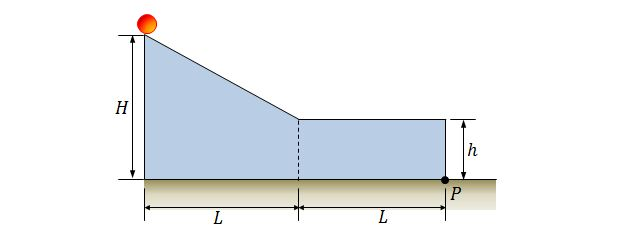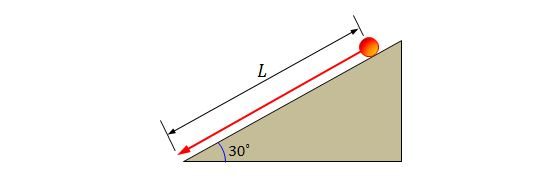Classical Mechanics

# Conservation of rotational and translational energyA solid ball rolls on a slope from rest starting from a height of $$H=7.0\text{ m}$$ and then rolls on a horizontal region, as shown in the above figure. The horizontal distance of the slope and the distance of the horizontal region are both equal to $$L=6 \text{ m},$$ and the height of the horizontal region is $$h=3.0\text{ m}.$$ Approximately how far horizontally from point $$P$$ does the ball hit the floor?

The rotational inertia of a solid sphere about any diameter is $$I=\frac{2}{5}MR^2,$$ where $$M$$ and $$R$$ are the mass and the radius of the solid sphere, respectively, and the gravitational acceleration is $$g=9.8\text{ m/s}^2.$$

A brand new off-road $$6 \times 6$$ pickup truck has six wheels. If the total mass of the pickup truck is $$1200\text{ kg}$$ and the mass of each of the six wheels is $$19\text{ kg},$$ what fraction of its total kinetic energy is due to the rotation of the wheels about their center axles?

Assume that each of the six wheels is a uniform disk.

A very thin hoop with a mass of $$180\text{ kg}$$ is rolling along a horizontal floor. If the speed of the hoop's center of mass is $$0.180\text{ m/s},$$ how much work must be done on the hoop to stop it?A solid cylinder with radius $$40\text{ cm}$$ and mass $$12\text{ kg}$$ is rolling down from rest without slipping for a distance of $$L=10.0\text{ m},$$ as shown in the above figure. The angle of the slope is $$\theta=30^\circ.$$ What is the approximate angular speed of the cylinder about its center when it just meets the bottom of the slope, assuming that the gravitational acceleration is $$g=9.8\text{ m/s}^2?$$

Consider a situation where a uniform solid sphere, which was rolling smoothly along a horizontal floor, rises up along a $$30.0^\circ$$ slope. If it stops after it has rolled $$9.00\text{ m}$$ along the slope and then begins to roll backward, what was its initial speed?

The rotational inertia of a solid sphere about any diameter is $$I=\frac{2}{5}MR^2,$$ where $$M$$ and $$R$$ are the mass and the radius of the solid sphere, respectively, and the gravitational acceleration is $$g=9.8\text{ m/s}^2.$$

×